# Math in Focus Grade 2 Chapter 5 Answer Key Multiplication and Division

Go through the Math in Focus Grade 2 Workbook Answer Key Chapter 5 Multiplication and Division to finish your assignments.

## Math in Focus Grade 2 Chapter 5 Answer Key Multiplication and Division

Math Journal

Look at the picture.

Put 12 stars into equal groups in different ways. What are the multiplication sentences and division sentences that you can write? Draw circles around the stars to help you.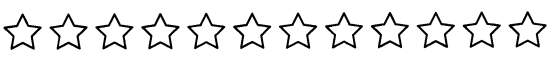Put on You? Thinking cap!

Challenging Practice

Maya is making silly stuffed animals. Study the number of eyes each stuffed animal has. Find a pattern. Then draw the eyes Maya will put on the last two stuffed animals.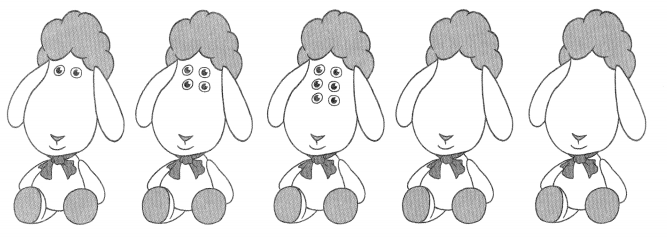Problem Solving

An open jar has 36 sugar cubes inside. Mighty Ant takes 4- days to carry all the sugar cubes back to his nest. He carries the same number of sugar cubes each day. How many sugar cubes does he carry each day?

I can solve this problem by drawing a picture or by acting it out with cubes.Chapter Review/Test

Vocabulary

Match.

Question 1.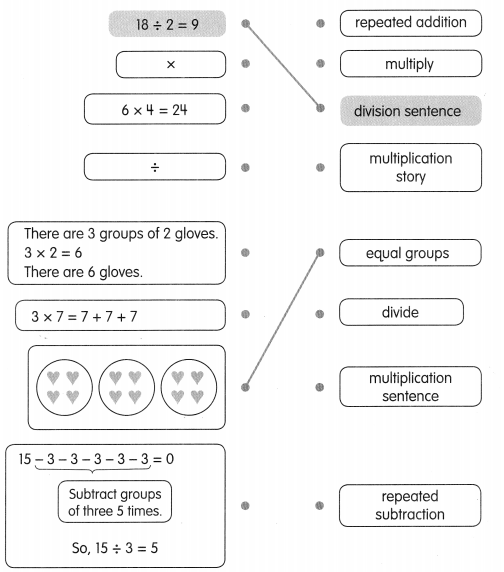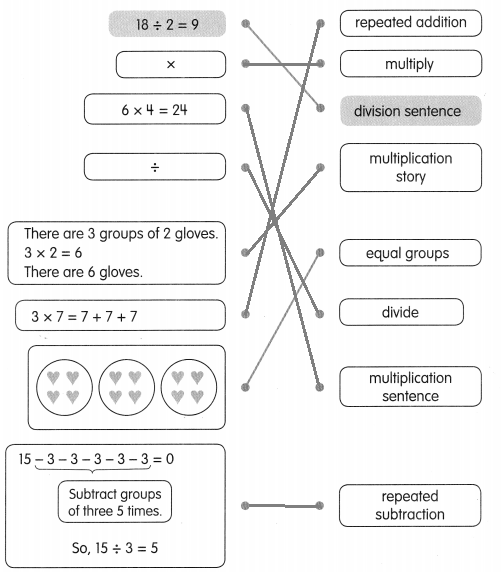Concepts and Skills

Fill in the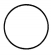with +, -, ×, or ÷.

Question 2.
6 groups of 2 = 62
6 groups of 2 = 6 × 2

Question 3.
84 = 32
8 multiply by 4 = 32
8 × 4 = 32

Question 4.
3 × 5 = 555
3 × 5 = 5 + 5 + 5

Question 5.
2044444 = 0
20 – 4 – 4 – 4 – 4 – 4 = 0

Question 6.
205 = 4
20 divided by 5 = 4
20 ÷ 5 = 4

Question 7.
153 = 5
15 divided by 3 = 5
15 ÷ 3 = 5

Find the missing numbers.
Question 8.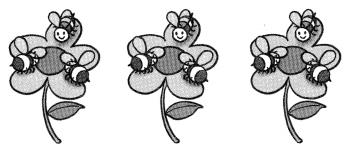3 × 3 = ___
There are ___ bees on the flowers in all.
3 × 3 = 9
There are 9 bees on the flowers in all.

Question 9.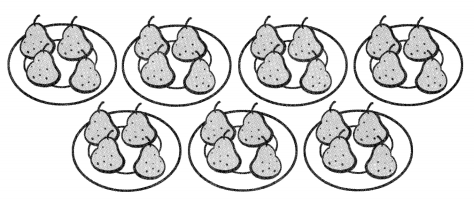7 × 4 = ___
There are ___ pears on the plates in all.
7 × 4 = 28
There are 28 pears on the plantes in all.

Question 10.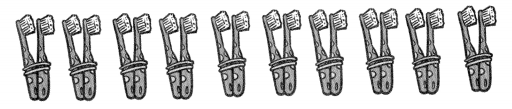10 × 2 = ___
There are ___ tooth brushes in all.
10 × 2 = 20
There are 20 tooth brushes in all.

Question 11.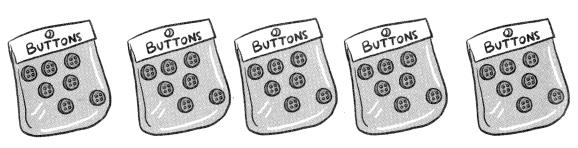5 × 7 = ___
There are ___ buttons in all.
5 × 7 = 35
There are 35 buttons in all.

Find the missing numbers.

Question 12.
Divide 8 bananas onto 4 plates equally.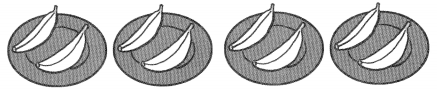8 ÷ 4 = ___
Given that
Number of bananas are 8
The 8 bananas are divided in the 4 plates.
Each plate contains = 8 ÷ 4 = 2
There are 2 bananas in each plate.

Question 13.
Divide 20 marbles into 5 equal groups.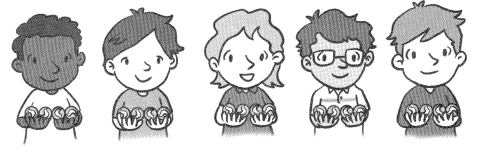20 ÷ 5 = ______
Given that
Number of marbles = 20
The 20 marbles are divided into 5 groups.
Each group contains = 20 ÷ 5 = 4
Therefore the each person has 4 marbles.

Question 14.
Divide 18 stamps into groups of 2.18 ÷ 2 = _______
Given that
Number of stamps are 18.
In 18 stamps every 2 stamps are divided into 1 group
18 ÷ 2 = 9
Therefore the 18 stamps are divided into 9 groups.

Question 15.
Divide 24 almonds into groups of 3.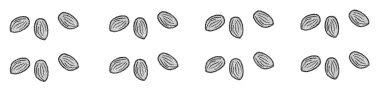24 ÷ 3 = ___
Given that
There are 24 almonds.
The 3 of almonds are divided into 1 group, So
24 ÷ 3 = 8
Therefore 24 almonds are divided into 8 groups.

Problem Solving

Solve.

Question 16.
Every day, Mr. Smith collects 3 eggs from his chickens. How many eggs does he collect in a week?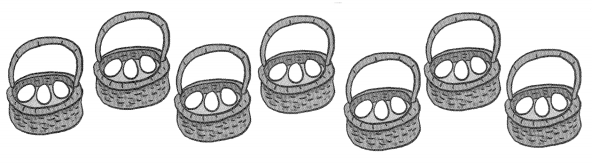Mr. Smith collects ____ eggs in a week.
Given that
Mr. Smith collects 3 eggs from his chicken every day.
Eggs collected in 1 week equal to
1 week = 7 days.
Therefore 7 days × 3 eggs = 21 days
Mr.Smith collects 27 eggs in a week.

Question 17.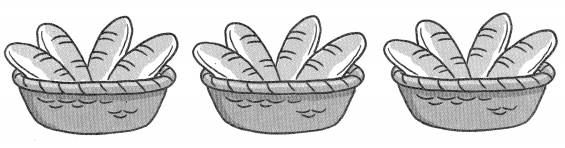There are ____ loaves of bread in all.
Given that
The number of baskets equal to 3.
Number of loaves of a bread is 3 × 4 = 12.
There are 12 loaves of bread in all.

Question 18.
Meg has 35 stickers. She has an album with 5 pages. She puts an equal number of stickers on each page. How many stickers are on each page?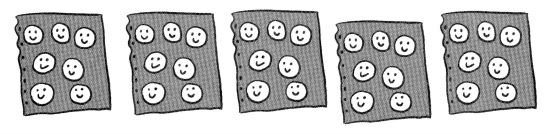There are ___ stickers on each page.
Given that
Meg has 35 stickers
She has a album with 5 pages and she puts equal number of stickers on each page.
Stickers on each page = 35 ÷ 5 = 7
There are 7 stickers on each page.

Question 19.
Mrs. Barker has 30 star stickers. She gives each student 5 star stickers. How many students are there?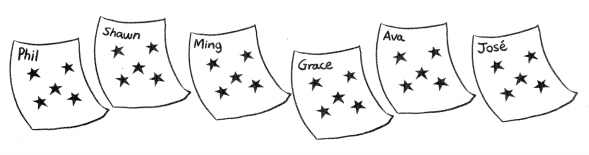There are ____ students.